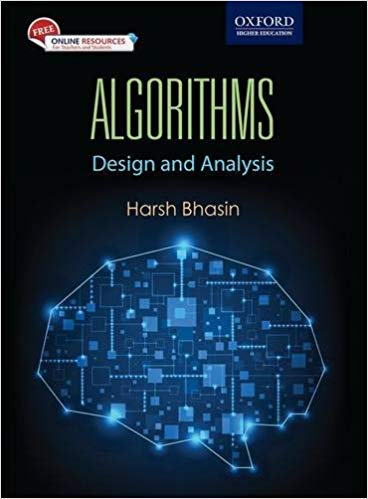## Recent Post

Algorithms: Design and Analysis of is a textbook designed for the undergraduate and postgraduate students of computer science engineering, information technology, and computer applications. It helps the students to understand the fundamentals and applications of algorithms.

The book has been divided into four sections: Algorithm Basics, Data Structures, Design Techniques and Advanced Topics. The first section explains the importance of algorithms, growth of functions, recursion and analysis of algorithms. The second section covers the data structures basics, trees, graphs, sorting in linear and quadratic time. Section three discusses the various design techniques namely, divide and conquer, greedy approach, dynamic approach, backtracking, branch and bound and randomized algorithms used for solving problems in separate chapters. The fourth section includes the advanced topics such as transform and conquer, decrease and conquer, number thoeretics, string matching, computational geometry, complexity classes, approximation algorithms, and parallel algorithms. Finally, the applications of algorithms in Machine Learning and Computational Biology areas are dealt with in the subsequent chapters. This section will be useful for those interested in advanced courses in algorithms.

The book also has 10 appendixes which include topics like probability, matrix operations, Red-black tress, linear programming, DFT, scheduling, a reprise of sorting, searching and amortized analysis and problems based on writing algorithms.

The concepts and algorithms in the book are explained with the help of examples which are solved using one or more methods for better understanding. The book includes variety of chapter-end pedagogical features such as point-wise summary, glossary, multiple choice questions with answers, review questions, application-based exercises to help readers test their understanding of the learnt concepts.

Buy :

PDF Download :